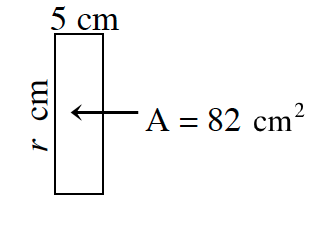### Home > CC1 > Chapter 5 > Lesson 5.2.2 > Problem5-65

5-65.

Find the missing side lengths of each rectangle, (a) and (c) or square (b) and (d). Homework Help ✎

1.  $x\ \text{cm}$ $100\ \text{cm}$ $\text{A}=500\ \text{cm}^2$

To find the area of a rectangle, multiply the length
by the width.

$(100\text{ cm})(x\text{ cm})=500\text{ cm}^2$

Solve for $x$.

1.  $y\ \text{cm}$ $\text{A}=$$\ \ \ \ 64\ \text{cm}^2$ $8\ \text{cm}$

Recall the properties of a square. All the side lengths must be equal.

Since one side is already given, you can conclude that the other side is also $8$ cm.

1.See the help for part (a).

$16.4$ cm

1.  $\ \text{A}=$$\ \ \ \ 25\ \text{cm}^2$ $w\ \text{cm}$ $5\ \text{cm}$

See the help for part (b).